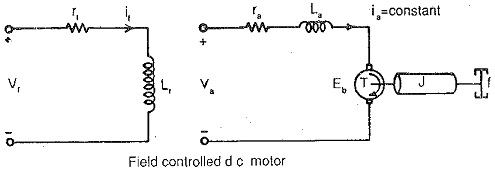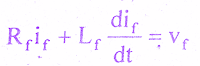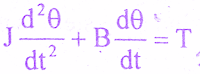# Transfer Function of Field Controlled DC Motor

0
181

#### The speed control system is an electro-mechanical control system.The electrical system consists of armature and field circuit but for analysis purpose, only field circuit is considered because the armature is excited by a constant voltage.The mechanical system consists of the rotating part of the motor and the load connected to the shaft of the motor.The field controlled DC motor speed control system is shown in the below figure.For this field controlled DC motor we shall find transfer function.#### The equivalent circuit of field is shown in the below figure.#### By Kirchoff ‘s voltage law, we can write#### The mechanical system of the motor is shown in the below figure.#### The differential equation governing the mechanical system of the motor is given by,#### The differential equations governing the field controlled DC motor are,in the derivation of transfer function of field controlled dc motor.#### Equating equations (2) & (3) we get,#### On substituting to If(s) from equation (4) in equation (5) we get,transfer function of field controlled dc motor.#### Tm = J/B     = Mechanical time constant

READ HERE  What is Commutation : Working Principle, Effects on DC Machines